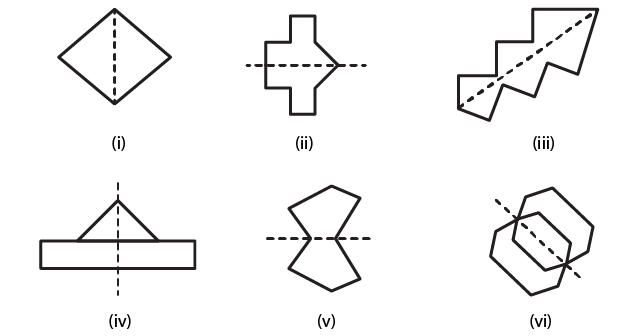# RD Sharma Solutions for Class 6 Maths Chapter 17: Symmetry Exercise 17.1

In our daily life, we come across many structures which are symmetrical in shape. When we cut those kinds of structures into two halves, we get identical parts on both sides. The line which divides a figure into two equal halves is called the line or axis of symmetry. Exercise 17.1 contains problems of RD Sharma textbook to help students get an idea about the concept of symmetry which are important from the exam point of view. Students who aspire to score well in the exam can use RD Sharma Solutions Class 6 Maths Chapter 17 Symmetry Exercise 17.1 PDF which are provided here.

## RD Sharma Solutions for Class 6 Maths Chapter 17: Symmetry Exercise 17.1 Download PDF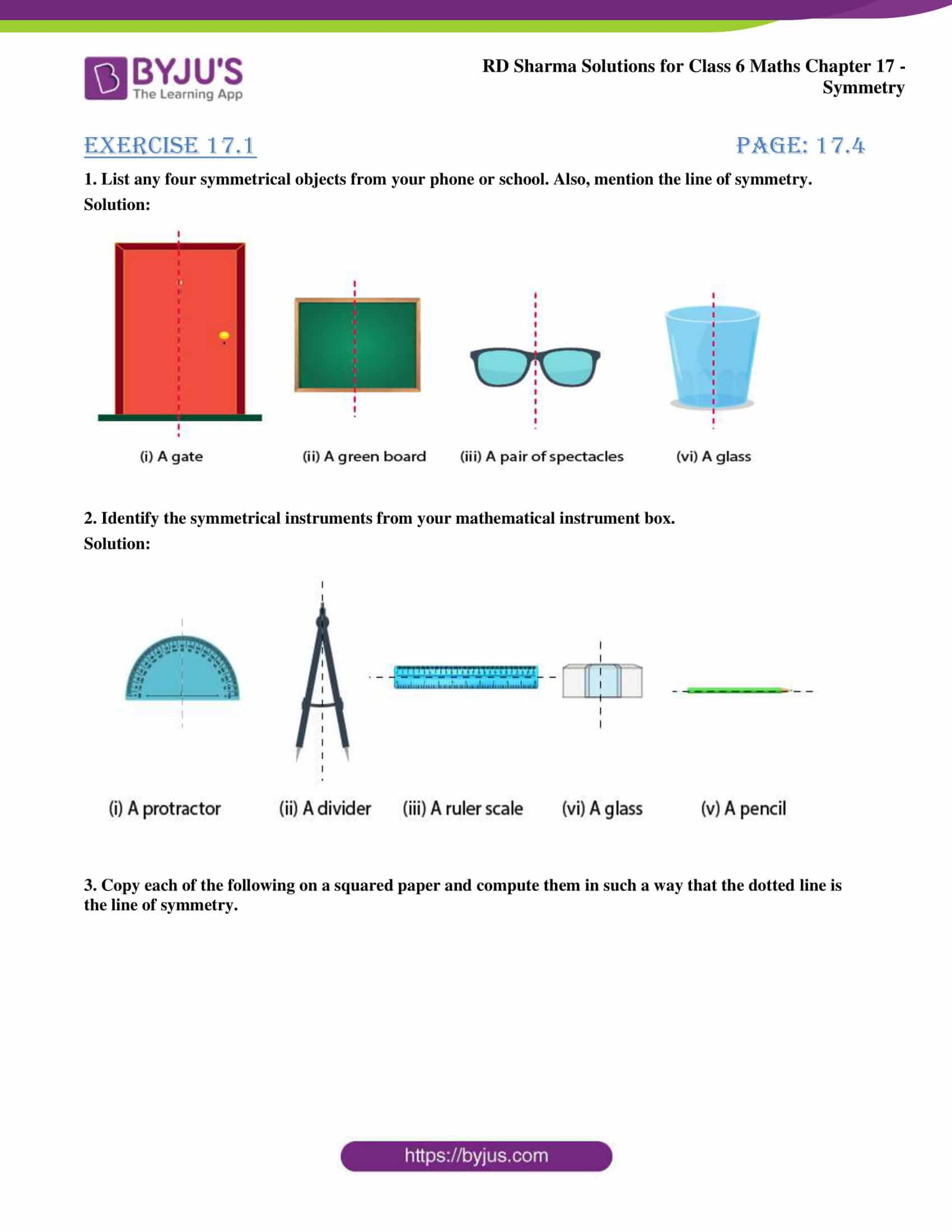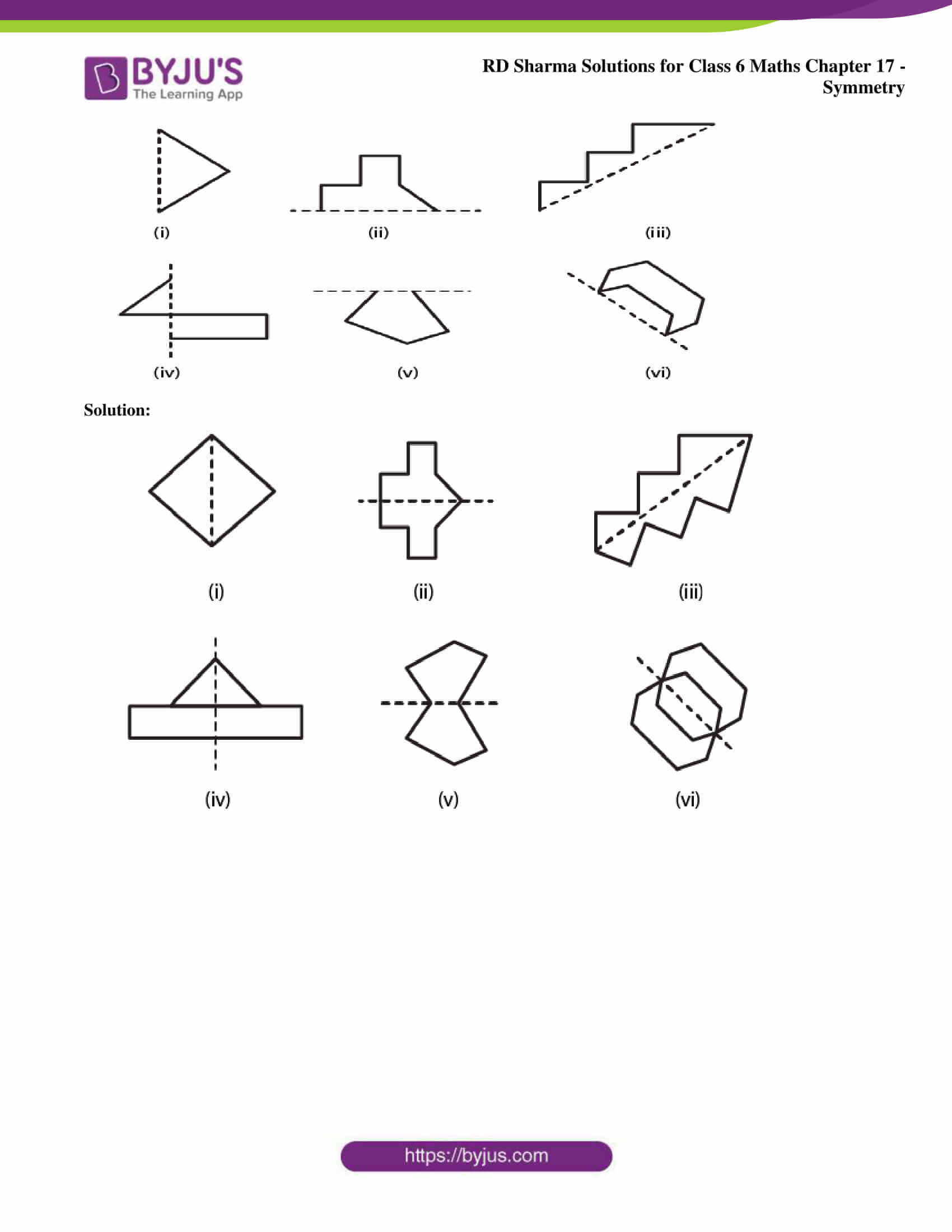### Exercise 17.1 page: 17.4

1. List any four symmetrical objects from your phone or school. Also, mention the line of symmetry.

Solution: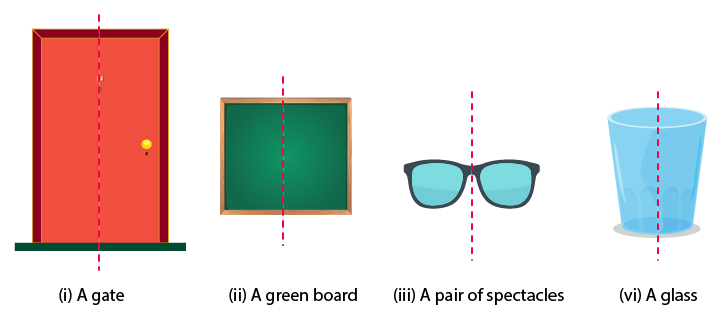2. Identify the symmetrical instruments from your mathematical instrument box.

Solution: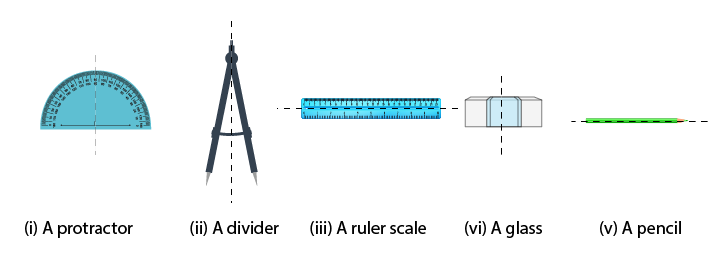3. Copy each of the following on a squared paper and compute them in such a way that the dotted line is the line of symmetry.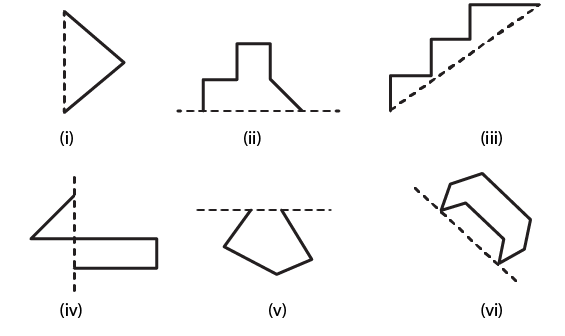Solution: